# Proof (logic)

In general, a proof is a demonstration that a specified statement follows from a set of assumed statements. The specified statement that follows from the assumed statements is called the conclusion of the proof and the assumed statements that the conclusion follows from are called the premises of the proof.

Particularly, in mathematics, a proof is a demonstration that the conclusion is a necessary consequence of the set of premises, i.e. the conclusion must be true if the premises are all true. Also, in logic, a proof is formally meant to be a sequence of formulas in some deductive system that shows the transformation from the set of premises (expressed as formulas) into the conclusion (also expressed as a formula) by the rules specified in the deductive system. The notion of proofs in this sense is a subject of the study in the field of proof theory.

There are various kinds of methods for proofs. The list of common methods are: direct proof, proof by induction, proof by transposition, proof by contradiction, nonconstructive proof, constructive proof, proof by exhaustion, probabilistic proof, combinatorial proof.

## Formal and Informal Proofs

In general, a proof is a demonstration that a specified statement follows from a set of assumed statements. The specified statement that follows from the assumed statements is called the conclusion of the proof and the assumed statements that the conclusion follows from are called the premises of the proof.

In mathematics, proofs are often expressed in natural language with some mathematical symbols. These type of proofs are called informal proof. A proof in mathematics is thus an argument showing that the conclusion is a necessary consequence of the premises, i.e. the conclusion must be true if all the premises are true. When all the premises of proofs are statements that have been previously agreed on for the purpose of the study in a given mathematical field, which are called axioms, the conclusions of such proofs are called theorems.

On the other hand, in logic, a proof is formally meant to be a sequence of formulas in some deductive system that shows the transformation from the set of premises (expressed as formulas) into the conclusion (also expressed as a formula) by the rules specified in the deductive system (called the rules of inference). When all the premises of proofs are axioms in the deductive system, i.e. the formulas syntactically specified in the deductive system, the conclusions of proofs are called theorems as in mathematics. Proof theory studies this notion of proof as its subject matter.

Although proofs can be written completely in a formal language, for practical reasons, proofs involves a natural language, such as English, and are often expressed as logically organized and clearly worded informal arguments intended to demonstrate that a formal symbolic proof can be constructed. Such arguments are typically easier to check than purely symbolic ones—indeed, many mathematicians would express a preference for a proof that not only demonstrates the validity of a theorem, but also explains in some way why it is obviously true. In some cases, a picture alone may be considered as sufficient to prove a theorem.

## Methods of proof

### Direct proof

In direct proof, the conclusion is established by logically combining the axioms, definitions, and earlier theorems. For example, direct proof can be used to establish that the sum of two even integers is always even:

For any two even integers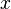$x$ and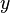$y$ we can write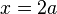$x=2a$ and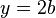$y=2b$ for some integers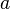$a$ and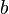$b$, since both$x$ and$y$ are multiples of 2. But the sum$x+y = 2a + 2b = 2(a+b)$ is also a multiple of two, so it is therefore even by definition.

This proof uses definition of even integers, as well as distribution law.

### Proof by induction

A proof by induction is a method to prove that a given property holds every element of a countable set, which is often identified with the set of natural numbers. Let N = {0, 1, 2, 3, 4, ... } be the set of natural numbers and P(n) be a mathematical statement involving the natural number n belonging to N. To prove by induction that P(n) hold of every n in N, we have only to prove the following two things:

• (i) P(1) is true, ie, P(n) is true for n = 1
• (ii) P(m + 1) is true whenever P(m) is true, ie, P(m) is true implies that

P(m + 1) is true.

### Proof by transposition

Proof by Transposition establishes the conclusion "if p then q" by proving the equivalent contrapositive statement "if not q then not p."

In proof by contradiction (also known as reductio ad absurdum, Latin for "reduction into the absurd"), it is shown that if some statement were false, a logical contradiction occurs, hence the statement must be true.

### Nonconstructive proof

A nonconstructive proof establishes that a certain mathematical object must exist (e.g. "Some X satisfies f(X)"), without explaining how such an object can be found. Often, this takes the form of a proof by contradiction in which the nonexistence of the object is proven to be impossible. In contrast, a constructive proof establishes that a particular object exists by providing a method of finding it.

### Constructive Proof

Constructive proof, or proof by example, is the construction of a concrete example with a property to show that something having that property exists. Joseph Liouville, for instance, proved the existence of transcendental numbers by constructing an explicit example. The field of mathematics which only allows constructive proofs is called constructive mathematics.

### Proof by exhaustion

In Proof by exhaustion, the conclusion is established by dividing it into a finite number of cases and proving each one separately. The number of cases sometimes can become very large. For example, the first proof of the four color theorem was a proof by exhaustion with 1,936 cases. This proof was controversial because the majority of the cases were checked by a computer program, not by hand. The shortest known proof of the four color theorem today still has over 600 cases.

### Probabilistic proof

A probabilistic proof is one in which an example is shown to exist by methods of probability theory—not an argument that a theorem is 'probably' true. The latter type of reasoning can be called a 'plausibility argument'; in the case of the Collatz conjecture it is clear how far that is from a genuine proof. Probabilistic proof, like proof by construction, is one of many ways to show existence theorems.

### Combinatorial proof

A combinatorial proof establishes the equivalence of different expressions by showing that they count the same object in different ways. Usually a bijection is used to show that the two interpretations give the same result.

## End of a proof

Sometimes, the abbreviation "Q.E.D." is written to indicate the end of a proof. This abbreviation stands for "Quod Erat Demonstrandum", which is Latin for "that which was to be demonstrated". An alternative is to use a small rectangle with its shorter side horizontal (), known as a tombstone or halmos.